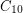## Weak saturation of the cube in the clique ★

Author(s): Morrison; Noel

Problem

Determine.

## Convex Equipartitions with Extreme Perimeter ★★

Author(s): Nandakumar

To divide a given 2D convex region C into a specified number n of convex pieces all of equal area (perimeters could be different) such that the total perimeter of pieces is (1) maximized (2) minimized.

Remark: It appears maximizing the total perimeter is the easier problem.

Keywords: convex equipartition

## Turán Problem for $10$-Cycles in the Hypercube ★★

Author(s): Erdos

Problem   Bound the extremal number ofin the hypercube.

Keywords: cycles; extremal combinatorics; hypercube